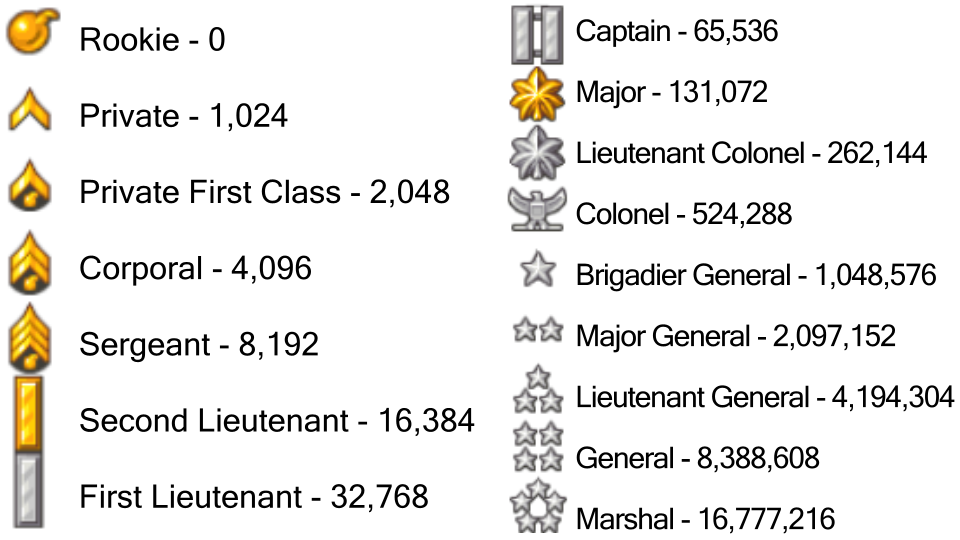#### Howdy, Stranger!

It looks like you're new here. If you want to get involved, click one of these buttons!

# Score/Rank Chart + Fun Math >:)I made this quick little cheat-sheet so you can see how much exp you need for next rankTo know when the next percentage is for your rank is, take the minimum exp required for your rank and multiply it by 1.xx. For example, the exp needed for your rank times 1.01 would be the amount of exp you need for 1 percent completion of your rank.

If that explanation was a little hard to follow, I'll do the work below for my rankI'm currently rank 11, Brigadier General. The minimum exp to have this rank is 1,048,576. If I wanted to find how much exp is needed to get to 50%, I simply multiply 1,048,576 by 1.5 (1048576 x 1.5) to get 1,572,864. My answer means that I would need a total of 1,572,864 exp to be 50% completed the rank. If you get a decimal, just round the number up (even if the decimal ends in something less than .5), as you're not able to have decimals for experience points.

If you wanted to find how close you are to the next rank, you could use two ways (one being more easier to visualize than the other, but both are interesting)

The first way is taking the amount of exp you currently have in total and dividing it by the minimum amount of exp needed to be your rank. I currently have 1,339,955 exp, and the minimum amount of exp needed for my rank is 1,048,576. I divide 1,339,955 by 1,048576 (1339955/1048576) to get an answer of 1.27788067. The important part is are the two digits after the one, 27. This matches up with the percentage I currently have. The cool part is that you can sort of approximate how close you are to the next percentage. If your answer is something like 1.01, that means you're exactly at one percent for your rank. If your answer is something like 1.0195, that means you are very very close to getting to 2%. This is useful if you want to figure out the percentages for other players as well, which I like to do sometimesThe other way is by subtracting your total exp from the total number of exp needed to have the next percentage for your rank. My current number is 1,339,955, and the next percentage for me is 28%. I multiply 1,048,576 by 1.28 first in order to find out how much exp is needed for 28%. The answer is 1342177.28, which I will round to 1,342,178. I then do 1,342,178 minus 1,339,955 (1342178 - 1339955) to get an answer of 2223. Not much exp until the next percent!You can also find the amount of exp it takes to rank up. First, you need to identify your rank and the minimum exp to get it. To change it up a bit, I'll use the Major rank for example. You need 131,072 total exp to achieve the Major rank. Then, you multiply that number by the next percentage up. In this case, I multiply 131,072 by 1.01 (131072 x 1.01), where 1.01 represents 1 percent ... which gives you the answer of 132382.72, which I'll round to 132,383. Finally, I subtract 132,383 which is the amount of exp needed for one percent and the minimum exp which is 131,072 (132383 - 131072) which gives the answer of 1311. 1311 represents how much score it takes to go up a percent.

One exception you may notice in the rank chart is the Rookie rank, where the minimum score is 0. No need to panic! To find each total exp, take the total exp needed for the Private rank (1,024) and multiply it by 0.xx. For example, 1,024 times 0.01 will give you how much score is needed to go up one percent. The answer is 10.24, which would be rounded up to 11. I think you got it from there!I hope that even through the walls of text you learned something! (assuming it made sense :^) )

if there's a problem, feel free to clarify in comments! I wrote this all in one go and I feel exhausted from all of the typing, so I wouldn't be surprised if I made a couple spelling errors (whoops!)

• niceI also have a similar chart which I just made for myself -• that's bc ur unqiue, @Gally Ps: were are you??? I don't see you any more in AU?